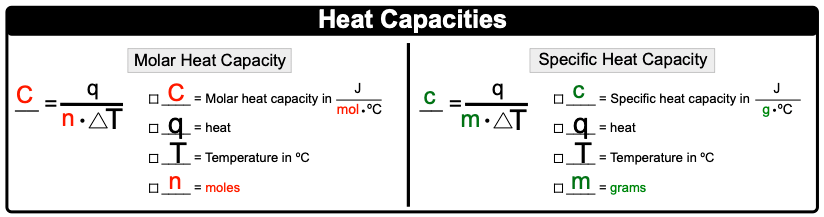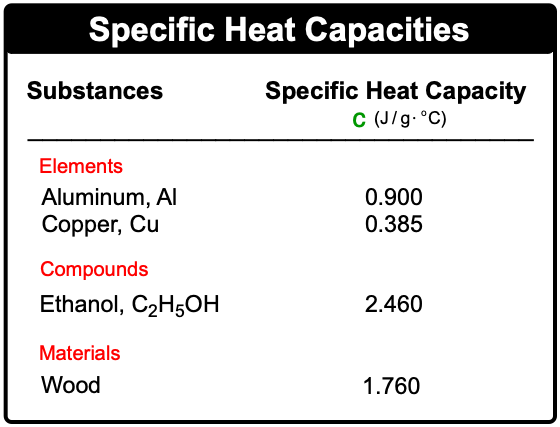Clutch Prep is now a part of Pearson
Ch.6 - Thermochemistry WorksheetSee all chapters

# Heat Capacity

See all sections
Sections
Nature of Energy
Kinetic & Potential Energy
First Law of Thermodynamics
Internal Energy
Endothermic & Exothermic Reactions
Heat Capacity
Constant-Pressure Calorimetry
Constant-Volume Calorimetry
Thermal Equilibrium
Thermochemical Equations
Formation Equations
Enthalpy of Formation
Hess's Law
Enthalpy (IGNORE)

Heat Capacity is the amount of heat required to change the temperature of a substance.

###### Understanding Heat Capacity

Concept #1: Heat CapacityExample #1: If the temperature of a water bath goes from 25 K to 50 K, what can be said about the amount of heat?

Concept #2: Molar & Specific Heat CapacityExample #2: If 15.7 g of silver raises its temperature by 17.2 ºC when it absorbs 6845.5 J, what is its molar heat capacity?

Concept #3: Rearranged Specific Heat FormulaExample #3: How much heat (in kJ) is released when 120.0 g H2O goes from 90 ºC to 45 ºC? The specific heat capacity of H2O is 4.184 J / g  ºC.

Practice: A sample of copper absorbs 3.53 kJ of heat, which increases the temperature by 25 ºC, determine the mass (in kg) of the copper sample if the specific heat capacity of copper is 0.385 J / g ºC.

Practice: Based on their given specific heat capacities which compound would show the greatest temperature change upon absorbing 25.0 J of heat?Practice: 50.00 g of heated metal ore is placed into an insulated beaker containing 822.5 g of water. Once the metal heats up the final temperature of the water is 32.08 ºC. If the metal gains 14.55 kJ of energy, what is the initial temperature of the water? The specific heat capacity of copper is 4.184 J / g ºC.